﻿ Modeling of the Scattering Process and the Optical Photo-generation Rate of a Dye Sensitized Solar Cell: Influence of the TiO<SUB>2</SUB> Radius

### Modeling of the Scattering Process and the Optical Photo-generation Rate of a Dye Sensitized Solar C...

E. H. O. Gueye, P. D. Tall, O. Sakho, C. B. Ndao, M. B. Gaye, N. M. Ndiaye, B. D. Ngom, A.C. Beye

American Journal of Nanomaterials

## Modeling of the Scattering Process and the Optical Photo-generation Rate of a Dye Sensitized Solar Cell: Influence of the TiO2 Radius

E. H. O. Gueye1, P. D. Tall1, O. Sakho1, C. B. Ndao1, M. B. Gaye1, N. M. Ndiaye1, B. D. Ngom1,, A.C. Beye1

1Groupe de physique du Solide et Sciences des Matériaux, Faculté des Sciences et Techniques Université Cheikh Anta Diop de Dakar (UCAD), B.P. 25114 Dakar-Fann Dakar (Sénégal)

### Abstract

We report on a methodology for optical and electrical modeling of dye-sensitized solar cells (DSSCs). In order to take into account the scattering process, the optical model is based on the determination of the effective permittivity of the mixture and the scattering coefficient using Mie and Bruggeman theories, considering spherical particles. Then, from the radiative transfer equation, the optical generation rate of cell is deduced. From the presented model, the dependence effects of the nanoparticles size upon the extinction coefficient and the optical generation rate are evidenced. Thus, we noticed that the extinction coefficient decreases with the increase of the TiO2 nanoparticles and vanishes when the wavelengths increases in the visible spectrum. A significant uniformity of the absorption for radius smaller than 10 nm is observed, however at a radius about 80 nm, we observe a non-uniformity. The simulated results based on this model are in good agreement with the experimental results.

• E. H. O. Gueye, P. D. Tall, O. Sakho, C. B. Ndao, M. B. Gaye, N. M. Ndiaye, B. D. Ngom, A.C. Beye. Modeling of the Scattering Process and the Optical Photo-generation Rate of a Dye Sensitized Solar Cell: Influence of the TiO2 Radius. American Journal of Nanomaterials. Vol. 4, No. 3, 2016, pp 58-62. http://pubs.sciepub.com/ajn/4/3/2
• Gueye, E. H. O., et al. "Modeling of the Scattering Process and the Optical Photo-generation Rate of a Dye Sensitized Solar Cell: Influence of the TiO2 Radius." American Journal of Nanomaterials 4.3 (2016): 58-62.
• Gueye, E. H. O. , Tall, P. D. , Sakho, O. , Ndao, C. B. , Gaye, M. B. , Ndiaye, N. M. , Ngom, B. D. , & Beye, A. (2016). Modeling of the Scattering Process and the Optical Photo-generation Rate of a Dye Sensitized Solar Cell: Influence of the TiO2 Radius. American Journal of Nanomaterials, 4(3), 58-62.
• Gueye, E. H. O., P. D. Tall, O. Sakho, C. B. Ndao, M. B. Gaye, N. M. Ndiaye, B. D. Ngom, and A.C. Beye. "Modeling of the Scattering Process and the Optical Photo-generation Rate of a Dye Sensitized Solar Cell: Influence of the TiO2 Radius." American Journal of Nanomaterials 4, no. 3 (2016): 58-62.

 Import into BibTeX Import into EndNote Import into RefMan Import into RefWorks

123
Prev Next

### 1. Introduction

Dye sensitized solar cells (DSSCs) are seen as an alternative to conventional solar cells and have been widely studied in recent years [1, 2, 3]. A DSSC is a mixture of nanostructured thin films, a sensitizer and an electrolyte containing the mediator sandwiched between two electrodes: a transparent conductive oxide as a transparent anode and a counter electrode as a cathode, such DSSC is depicted in Figure 1.Download asVeiw figureFigures index
Figure 1. Structure of the Dye sensitized solar cell

The efficiency of the dye absorption is one of the paramount parameters that determine the cell performance. Accordingly, the peculiar composition of the photoactive layer, makes it very complicate to establish an optoelectronic model for the DSSCs. Indeed, it is composed of a mixture of three components (titanium dioxide (TiO2), dye molecules and the electrolyte in which tri-iodide ions are immerged) which could raise homogeneity problems.

Nevertheless, various optical [7, 8], electrical [9-18] and optoelectronic [19, 20] models have been developed. But, one can notice that various phenomena such as molecular processes for the excitation of the dye, injection within the titanium dioxide and recombination of charges in the dye, cannot be simulated as it have been done for the conventional silicon solar cells . In this paper, an optical model of dye-sensitized solar cell will be presented, putting a focus on the accurate description of the optics in the photoactive layer (by applying Bruggeman's theory, Mie's Theory and radiative transfer equations). The influence of the TiO2 radius is also studied.

### 2. Computational Method

The model considered is depicted in Figure 2. Thus, our study is essentially limited to the photoactive layer sandwiched between the two electrodes. The photoactive layer is a mixture of Titanium dioxide (TiO2), a dye (Z907), a mediator and an electrolyte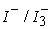. For the calculation of the effective permittivity, we use the same approach as that used in our previous paper .Download asVeiw figureFigures index
Figure 2. Schematic of the cell

Firstly, we calculate the scattering cross sections and absorption through the Mie theory in the case of a mono-dispersed nanoparticle size. Our medium is composed of three compounds; we calculate the scattering cross sections for the nanostructured thin films (TiO2 here) and electrolyte / mediator. The particle sizes of dyes are low , therefore they do not participate in the scattering process. Then macroscopic scattering and absorption coefficient s and k will be calculated for each compound, using the following equations.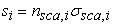(1)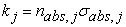(2)

Where i = electrolyte/mediator or TiO2; j = TiO2 or dye or electrolyte /mediator. The particle density of the electrolytic/ mediator will be given by the equation: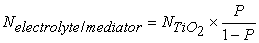(3)

The hypothesis we making here is to consider the TIO2/Dye as one particle. Since the diffusion of the dyes particles is very low we consider that the particle (TiO2/Dye) have the same dimensions as the simple TiO2 particle . On the other hands since the TiO2 particle doesn't absorb we consider also that the absorption of the particle (TiO2/Dye is just coming from the simple dye particle Therefore we have: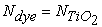(4)

With P the porosity.

Then: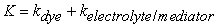(5)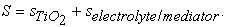(6)

Afterwards the macroscopic coefficients K and S are used to calculate the collimated flux (Ic and Jc) and diffused flux (Id and Jd). Basically, the approximation of the radiative transfer equation are done using four flux  and the equations are given by (equations: 7-10). These different flux are illustrated in Figure 3. In fact, the variation of the flows in the medium with an infinitesimal value of the thickness dx can be established using the equilibrium between the scattering and the absorption energy in forward and backward.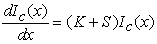(7)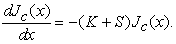(8)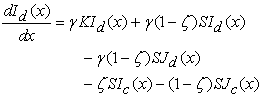(9)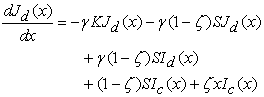(10)Download asVeiw figureFigures index
Figure 3. Schema of the cell with different flux

The factor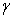is the equivalent path length for the diffuse flux which ranges from 1 for collimated light to 2 for isotropic scattered light . Thus, the analytical expression of the forward scattering ratio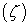is obtained from C. Rozé et al. analysis .

The four flux Ic, Id, Jc and Jd included in the previous equations, (7), (8), (9) and (10) are determined by taking into account the following boundary conditions.

At the Glass/Mixed zone: x=a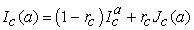(11)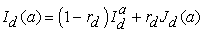(12)

where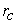,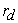,,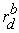the reflective coefficient of collimated beam, the reflective coefficient of radiation diffuse beam, the reflective coefficient of collimated beam at the back, the reflective coefficient of radiation diffuse beam at back side respectively.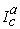and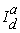are the incident collimated flux and the incident diffuse flux at the interface x=a.can be determined by taking into account the interference effects at the glass/mixed zone and air/glass interfaces and is given as: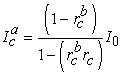(13)

In fact,is equal to zero and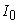is the incoming light.

At the Mixed zone/Glass: x=0

By considering the reflection effects at the air/glass and the glass/mixed interfaces the following conditions can be established: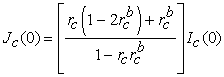(14)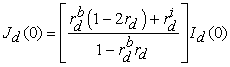(15)

Where: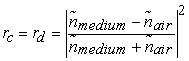(16)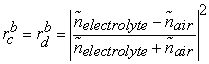(17)

Where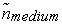is determined using bruggeman theory.

Thus, the local optical absorption rate per unit volume  can be established using the expression below: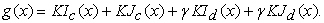(18)

Hence, the optical photons absorption rate is deduced from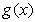, and is expressed as: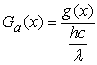(19)

And Gdye is deduced by: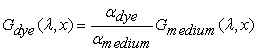(20)

Finally, the optical photo-generation rate of the DSSC is given by: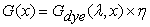(21)

where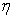being the injection rate of electrons.

### 3. Results and Discussion

The analytical model presented above is validated with experimental data reported in the literature (Cf section D) . After calculating the analytical new expressions presented in the previous section, a numeric based code program implemented by the commercial package Matlab software is established. Basically, numerical results are presented and discussed in this section. Thus, the macroscopic parameters such as the extinction coefficient, the four flux and the optical generation rate of the DSSC in term of various phenomenological parameters such as the wavelength of the incoming light, the TiO2 nanoparticles radius, the depth of the photoactive layer are depicted.

3.1. The Extinction Coefficient of the Mixture Material within the Photoactive Layer

The Figure 4 shows the extinction coefficient of the TiO2-dye (Z907) as a function of the wavelength for different TiO2 nanoparticles radius ranging from 10 and 20 nm. All the obtained spectrums show the same trend, however, when the radius increases, the extinction coefficient decreases. The reason is that, when the radius increases, the absorption decreases because of the disappearance of the surface absorption effects .

In Figure 5 is depicted the effective extinction coefficient of the mixture {TiO2+dye (Z907)+electrolyte+mediator} within the photoactive layer as function of the wavelength from 400-800 nm for different TiO2 nanoparticles radius ranging from 10 and 20 nm. Compared to the results obtained in figure 4, the intensity of the extinction coefficient presented in figure 5 decreases when the size of TiO2 nanoparticles increases. Three areas are identified: 400 nm to 450 nm, 450 nm to 600 nm and 600 nm to 800 nm. Except the wavelength region between 400 nm and 450 nm, where we observe a behavior similar to that of the mediator, the trend of the mixture is the same for the two other regions and completely different from the trend of the mediator. Such observed behavior on {TiO2+dye (Z907)+electrolyte+mediator} mixture on the first region is due to the absorbance of the mediator which happens only between 400 nm and 450 nm.Download asVeiw figureFigures index
Figure 4. Extinction coefficient of photo-active layer (mixture TiO2 + Z907) as a function of wavelength for different radii of the TiO2 particleDownload asVeiw figureFigures index
Figure 5. Extinction coefficient of photo-active layer (mixture TiO2 + Z907+acétonitrile-I-/I3-) as a function of wavelength for different radii of the TiO2 particle
3.2. Scattering of Multiple Particles

The values of the 4 flux Ic, Jc, Id and Jd can be calculated as function of the depth of the cell, from the diffusion coefficients, the macroscopic absorption, the forward scattering ratio and the equivalent path length. Figure 6 shows the flux as function of the cell depth. One can see that for small radius, Ic decreases slightly and much part of flux reaches the edge of the cell. This is due to the small extinction and diffusion coefficient for small radius. The others flux are very small compared to the Ic flux for 10 nm. This phenomenon became different with radius ranging from 20 nm to 40 nm, where Ic rapidly decreases, while Id increases in the first half of the cell. Therefore the light reaching the edge of the cell is spread. Jd has also a large value like Id. This due to the small increase in the diffusion coefficient. For much larger radius (80 nm), Ic rapidly decreases, while Id increases right at the edge before rapidly decreasing too. Because of the high diffusion of the molecules with larger radius, only a small part of the flux touches the edge of the cell.

3.3. Determination of the Generation Rate

Figure 7a shows, the generation rate of the photoactive layer depending on the depth of the mixed. In this figure, we compare our model and that of Wenger et al. . We can see that our results are in the same range of values than those calculated by Wenger et al. .

Figure 7b shows the generation rate of the photoactive layer as a function of depth and for different radius. It seems that for low radius the absorption is fairly homogeneous, as the scattering of light is low, as shown in Figure 6a, the intensity of the collimated light is almost constant. More the radius increases more scattering increases, which cause an increase of diffusion flux and a decrease of the collimated flux. Such behavior makes the major part of the intensity being absorbed at the edge of the cell, because of the process of scattering and extinction.Download asVeiw figureFigures index
Figure 6. Collimated flux (Ic, Jc) and diffuse flux (Id, Jd), for different radii of TiO2 (a − 10nm, b − 20nm, c − 40nm, d- 80nm) particle I0=1000W m–2Download asVeiw figureFigures index
Figure 7. Generation rate of photo active layer as a function of the cell depth (a: comparison between our model and Sophie Wenger results, b: for different radii of TiO2)

### 4. Conclusion

Our model allows us to see the influence of the nanoparticle size of TiO2 on the effective extinction coefficient of the mixture. The influence of the nanoparticle size of TiO2 on the generation rate was demonstrated. This study allowed seeing that for the small radius, the generation rate is homogeneous. When radius increases, almost all the light is absorbed at the edge of the cell. Our results are in agreement with those in the literature. However, this model shows limitations: the porosity is fixed, the nanoparticles are not ideally spherical and same size, and the difference between the reflected coefficient diffused and collimated could be taken into account for better precision.

### Acknowledgement

The authors are grateful for the funding by the UEOMA under the PAIS project.

### References

  O’Regan, B., Grätzel, M., “A low-cost, high-efficiency solar cell based on dye-sensitized colloidal TiO2 films,” Nature, 353-737, 1991.In article View Article  Wang, Q., Ito, S., Gratzel, M., Fabregat-Santiago, F., Mora-Sero, I., Bisquert, J., Bessho, T., and Imai, H., “Characteristics of High Efficiency Dye-Sensitized Solar Cells,” J. Phys. Chem. B 110, 25210-25221, 2006.In article View Article  PubMed  Mathew, S., Yella, A., Gao, P., Humphry-Baker, R., Curchod, B.F.E., Tavernelli, I., Rothlisberger, U., Nazeeruddin M.K., and Grätzel, M., “Dye-sensitized solar cells with 13% efficiency achieved through the molecular engineering of porphyrin sensitizers,” Nature Chemistry 6, 242-247, 2014.In article View Article  PubMed  Green, M. A., Emery, K., Hishikawa, Y., Warta, W., and Dunlop, E. D., “Solar cell efficiency tables (Version 45),” Progress in photovoltaics: research and applications, 23(1), 1-9, 2015.In article View Article  Chiba, Y.; Islam, A.; Watanabe, Y.; Komiya, R.; Koide, N.; Han, L., J. Appl. Phys., Part 2, 45, L638-L640, 2006.In article View Article  Gao, F.; Wang, Y., Shi, D., Zhang, J., Wang, M. K., Jing, X. Y., Humphry-Baker, R., Wang, P., Zakeeruddin, S. M., Grätzel, M., J. Am. Chem. Soc. 130, 10720-10728 ,2008.In article View Article  PubMed  Ferber, J., and Luther, J., “Computer simulations of light scattering and absorption in dye-sensitized solar cells.” Solar Energy Materials and Solar Cells 54 (1998)In article View Article  Rothenberger, G., Comte, P., Gratzel, M., “A contribution to the optical design of dye_sensitized nanocrystalline solar cells,” Solar Energy Materials & Solar Cells, 58, 321-336, 1999.In article View Article  Soedergren, S., Hagfeldt, A., Olsson, J., and Lindquist, S. E., “Theoretical models for the action spectrum and the current-voltage characteristics of microporous semiconductor films in photoelectrochemical cells,” The Journal of Physical Chemistry, 98(21), 5552-5556, 1994.In article View Article  Matthews, D., Infelta, P., and Grätzel, M, “Calculation of the photocurrent-potential characteristic for regenerative, sensitized semiconductor electrodes.” Solar Energy Materials and Solar Cells, 44(2), 119-155, 1996.In article View Article  Ferber, J., Stangl, R., and Luther, J., “An electrical model of the dye-sensitized solar cell,” Solar Energy Materials and Solar Cells, 53(1), 29-54, 1998.In article View Article  Usami, A., “Theoretical study of application of multiple scattering of light to a dye-sensitized nanocrystalline photoelectrichemical cell,” Chemical Physics Letters, 277(1), 105-108, 1997.In article View Article  Usami, A., “Theoretical study of charge transportation in dye-sensitized nanocrystalline TiO2 electrodes,” Chemical physics letters, 292(1), 223-228, 1998.In article View Article  Ferber, J., Stangl, R., and Luther, J., “An electrical model of the dye-sensitized solar cell,” Solar Energy Materials and Solar Cells, 53(1), 29-54, 1998.In article View Article  Stangl, R., Ferber, J., & Luther, J., “On the modeling of the dye-sensitized solar cell,” Solar Energy Materials and Solar Cells, 54(1), 255-264, 1998.In article View Article  Usami, A., & Ozaki, H., “Computer simulations of charge transport in dye-sensitized nanocrystalline photovoltaic cells,” The Journal of Physical Chemistry B, 105(20), 4577-4583, 2001.In article View Article  Bisquert, J., Cahen, D., Hodes, G., Rühle, S., & Zaban, A., “Physical chemical principles of photovoltaic conversion with nanoparticulate, mesoporous dye-sensitized solar cells,” The Journal of Physical Chemistry B, 108(24), 8106-8118, 2004.In article View Article  Filipič, M., Berginc, M., Smole, F., & Topič, M., “Analysis of electron recombination in dye-sensitized solar cell,” Current Applied Physics, 12(1), 238-246, 2012.In article View Article  Wenger, S., Schmid, M., Rothenberger, G., Gentsch, A., Gratzel, M., and Schumacher, J. O., “Coupled Optical and Electronic Modeling of Dye-Sensitized Solar Cells for Steady-State Parameter Extraction,” J. Phys. Chem. C 115, 10218–10229, 2011.In article View Article  Topič, M., Čampa, A., Filipič, M., Berginc, M., Krašovec, U. O., & Smole, F., “Optical and electrical modelling and characterization of dye-sensitized solar cells,” Current Applied Physics, 10(3), S425-S430, 2010.In article View Article  Gueye, E.H.O., Tall, P. D., Ndao, C. B., Dioum, A., Dione, A. N., & Beye, A. C. (2016). An Optical and Electrical Modeling of Dye Sensitized Solar Cell: Influence of the Thickness of the Photoactive Layer. American Journal of Modeling and Optimization, 4(1), 13-18.In article  C. Rozé, T. Girasole, G. Gréhan, G.Gouesbet, B.Maheu. Four-flux models to solve the scattering transfer equation in terms of Lorenz-Mie parameters. Optics communications 194. (2001). 251-263.In article View Article  G. Kortum, Reflectance Spectroscopy, Springer, Berlin, 1969.In article View Article  PubMed  B. Maheu, J. N. Letoulouzan and G. Gouesbet. Four-flux models to solve the scattering transfer equation in terms of Lorenz-Mie parameters. Applied Optics Vol. 23, No. 19 (1984).In article View Article  PubMed  A. Dioum, S. Ndiaye, E. H. O. Gueye, M. B. Gaye, D. N. Faye, O. Sakho, M. Faye and A. C. Beye. 3-D Modeling of bilayer heterojunction organic solar cell based on Copper Phthalocyanine and Fullerene (CuPc/C60): evidence of total excitons dissociation at the donor-acceptor interface. Global Journal of Pure and Applied Sciences, Vol 19 (2013).In article